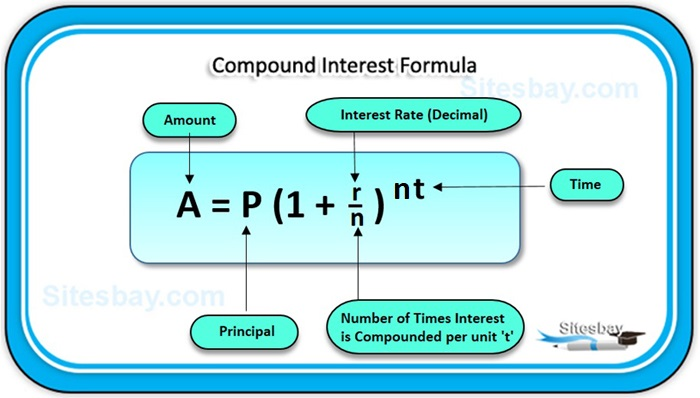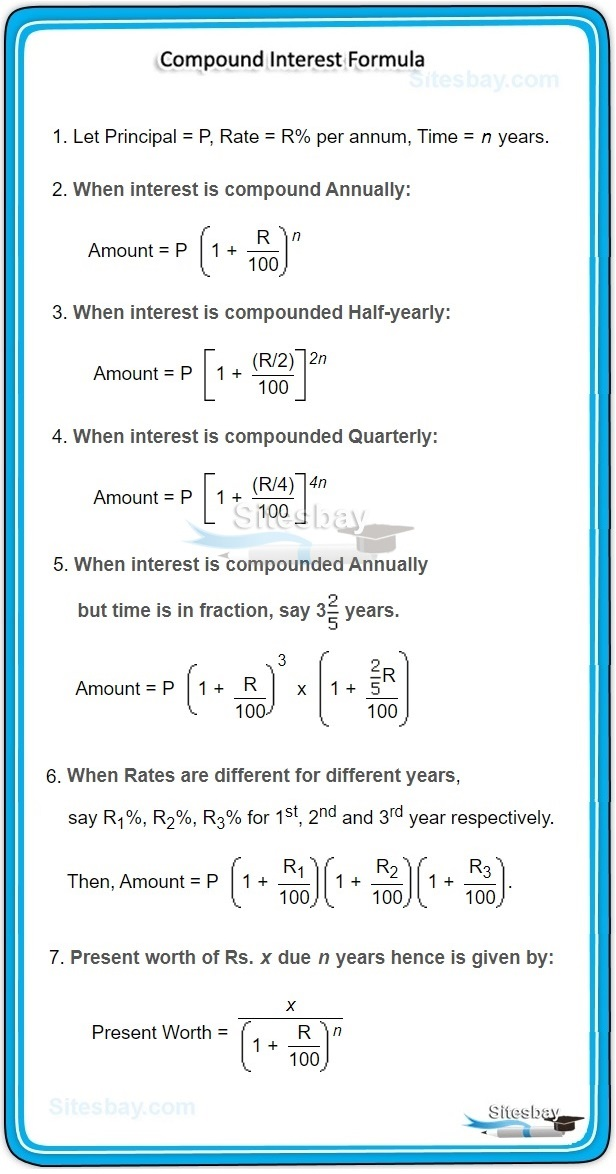# Compound Interest Problems

## Compound Interest Tips and Tricks

In a simple word Compound Interest is the interest on interest. The addition of interest to the principal sum each year or some fixed time period is known as compounding. The interest of each year or some fixed time period is added to the principal sum and the new amount becomes the principal for the next year and interest is calculated on the increased amount for the next year.## Formula

```A = P (1 + r/n) nt
```
• A = the future value of the investment/loan, including interest
• P = the principal investment amount (the initial deposit or loan amount)
• r = the annual interest rate (decimal)
• n = the number of times that interest is compounded per unit t
• t = the time the money is invested or borrowed forQuestion: If an amount of \$6,000 is deposited into a savings account at an annual interest rate of 4%, compounded monthly, the value of the investment after 5 years can be calculated as follows.

## Example

```P = 6000.
r = 4/100 = 0.04 (decimal).
n = 12
t = 5

If we plug those figures into the formula, we get the following:

A = 6000 (1 + 0.05 / 12) (12 * 5) = 7325.98

So, the investment balance after 10 years is \$7325.98
```